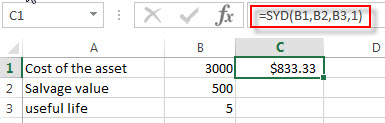## Excel SYD Function

This post will guide you how to use Excel SYD function with syntax and examples in Microsoft excel.

### Description

The Excel SYD function calculates the sum-of-years’digits depreciation of an asset for a specified period. And it will return a numeric value.

The SYD function is a build-in function in Microsoft Excel and it is categorized as a Financial Function.

The SYD function is available in Excel 2016, Excel 2013, Excel 2010, Excel 2007, Excel 2011 for Mac.

### Syntax

The syntax of the SYD function is as below:

`=SYD(cost, salvage, life, per)`

Where the SYD function arguments are:

• Cost -This is a required argument. The initial cost of the asset.
• Salvage -This is a required argument. The value of the asset at the end of the depreciation.
• Life -This is a required argument. The number of periods over which the asset is to be depreciated.
• Per -This is a required argument. The period that you want to calculate the depreciation for.

### Excel SYD Function Examples

The below examples will show you how to use Excel SYD Function to calculate the sum-of-years’digits depreciation for a period in the lifetime of an asset.

#1 to get the depreciation for the first year for an asset that cost 3000, with a salvage value of 500, and the useful life of the asset is 5, using the following formula:

`=SYD(B1,B2,B3,1) `### Related Functions

• Excel SLN Function
The Excel SLN function calculates the depreciation of an asset for one period based on the straight line depreciation. And it will return a numeric value.The syntax of the SLN function is as below:= SLN (cost, salvage, life)…
• Excel VDB Function
The Excel VDB function calculates the depreciation of an asset for a specified period based on the double declining balance method. And it will return a numeric value.The syntax of the VDB function is as below:= VDB (cost, salvage, life, start_period, end_period, [factor], [no_switch])…